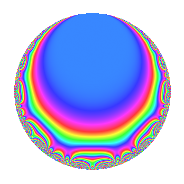# Properties

 Label 6024.2.a.jLevel 6024 Weight 2 Character orbit 6024.a Self dual Yes Analytic conductor 48.102 Analytic rank 1 Dimension 3 CM No Inner twists 1

# Related objects

## Newspace parameters

 Level: $$N$$ = $$6024 = 2^{3} \cdot 3 \cdot 251$$ Weight: $$k$$ = $$2$$ Character orbit: $$[\chi]$$ = 6024.a (trivial)

## Newform invariants

 Self dual: Yes Analytic conductor: $$48.1018821776$$ Analytic rank: $$1$$ Dimension: $$3$$ Coefficient field: 3.3.148.1 Coefficient ring: $$\Z[a_1, \ldots, a_{5}]$$ Coefficient ring index: $$2$$ Fricke sign: $$1$$ Sato-Tate group: $\mathrm{SU}(2)$

## $q$-expansion

Coefficients of the $$q$$-expansion are expressed in terms of a basis $$1,\beta_1,\beta_2$$ for the coefficient ring described below. We also show the integral $$q$$-expansion of the trace form.

 $$f(q)$$ $$=$$ $$q$$ $$+ q^{3}$$ $$+ \beta_{2} q^{5}$$ $$-3 q^{7}$$ $$+ q^{9}$$ $$+O(q^{10})$$ $$q$$ $$+ q^{3}$$ $$+ \beta_{2} q^{5}$$ $$-3 q^{7}$$ $$+ q^{9}$$ $$+ ( \beta_{1} + \beta_{2} ) q^{11}$$ $$+ ( -2 + \beta_{1} - \beta_{2} ) q^{13}$$ $$+ \beta_{2} q^{15}$$ $$+ ( 2 - 2 \beta_{1} - \beta_{2} ) q^{17}$$ $$+ ( 1 - \beta_{2} ) q^{19}$$ $$-3 q^{21}$$ $$-\beta_{2} q^{23}$$ $$+ ( 2 - \beta_{1} - \beta_{2} ) q^{25}$$ $$+ q^{27}$$ $$+ ( -1 - \beta_{1} - 2 \beta_{2} ) q^{29}$$ $$+ ( -1 - 2 \beta_{1} ) q^{31}$$ $$+ ( \beta_{1} + \beta_{2} ) q^{33}$$ $$-3 \beta_{2} q^{35}$$ $$+ ( -3 + \beta_{1} + 2 \beta_{2} ) q^{37}$$ $$+ ( -2 + \beta_{1} - \beta_{2} ) q^{39}$$ $$+ ( -2 + 3 \beta_{1} ) q^{41}$$ $$+ ( 5 - 2 \beta_{1} + \beta_{2} ) q^{43}$$ $$+ \beta_{2} q^{45}$$ $$+ ( 4 - 3 \beta_{1} - \beta_{2} ) q^{47}$$ $$+ 2 q^{49}$$ $$+ ( 2 - 2 \beta_{1} - \beta_{2} ) q^{51}$$ $$+ ( -7 + 2 \beta_{1} + \beta_{2} ) q^{53}$$ $$+ ( 6 + \beta_{1} - \beta_{2} ) q^{55}$$ $$+ ( 1 - \beta_{2} ) q^{57}$$ $$+ ( -5 - 2 \beta_{1} - \beta_{2} ) q^{59}$$ $$+ ( 1 - 2 \beta_{1} - \beta_{2} ) q^{61}$$ $$-3 q^{63}$$ $$+ ( -8 + 3 \beta_{1} - \beta_{2} ) q^{65}$$ $$+ ( 1 + 5 \beta_{1} - 3 \beta_{2} ) q^{67}$$ $$-\beta_{2} q^{69}$$ $$+ ( -11 - \beta_{2} ) q^{71}$$ $$+ ( -7 - 4 \beta_{1} + 2 \beta_{2} ) q^{73}$$ $$+ ( 2 - \beta_{1} - \beta_{2} ) q^{75}$$ $$+ ( -3 \beta_{1} - 3 \beta_{2} ) q^{77}$$ $$+ ( -1 + \beta_{1} + \beta_{2} ) q^{79}$$ $$+ q^{81}$$ $$+ ( -2 \beta_{1} - \beta_{2} ) q^{83}$$ $$+ ( -5 - 3 \beta_{1} + 3 \beta_{2} ) q^{85}$$ $$+ ( -1 - \beta_{1} - 2 \beta_{2} ) q^{87}$$ $$+ ( -2 + 3 \beta_{1} - 3 \beta_{2} ) q^{89}$$ $$+ ( 6 - 3 \beta_{1} + 3 \beta_{2} ) q^{91}$$ $$+ ( -1 - 2 \beta_{1} ) q^{93}$$ $$+ ( -7 + \beta_{1} + 2 \beta_{2} ) q^{95}$$ $$+ ( -3 + 6 \beta_{1} + \beta_{2} ) q^{97}$$ $$+ ( \beta_{1} + \beta_{2} ) q^{99}$$ $$+O(q^{100})$$ $$\operatorname{Tr}(f)(q)$$ $$=$$ $$3q$$ $$\mathstrut +\mathstrut 3q^{3}$$ $$\mathstrut +\mathstrut q^{5}$$ $$\mathstrut -\mathstrut 9q^{7}$$ $$\mathstrut +\mathstrut 3q^{9}$$ $$\mathstrut +\mathstrut O(q^{10})$$ $$3q$$ $$\mathstrut +\mathstrut 3q^{3}$$ $$\mathstrut +\mathstrut q^{5}$$ $$\mathstrut -\mathstrut 9q^{7}$$ $$\mathstrut +\mathstrut 3q^{9}$$ $$\mathstrut +\mathstrut 2q^{11}$$ $$\mathstrut -\mathstrut 6q^{13}$$ $$\mathstrut +\mathstrut q^{15}$$ $$\mathstrut +\mathstrut 3q^{17}$$ $$\mathstrut +\mathstrut 2q^{19}$$ $$\mathstrut -\mathstrut 9q^{21}$$ $$\mathstrut -\mathstrut q^{23}$$ $$\mathstrut +\mathstrut 4q^{25}$$ $$\mathstrut +\mathstrut 3q^{27}$$ $$\mathstrut -\mathstrut 6q^{29}$$ $$\mathstrut -\mathstrut 5q^{31}$$ $$\mathstrut +\mathstrut 2q^{33}$$ $$\mathstrut -\mathstrut 3q^{35}$$ $$\mathstrut -\mathstrut 6q^{37}$$ $$\mathstrut -\mathstrut 6q^{39}$$ $$\mathstrut -\mathstrut 3q^{41}$$ $$\mathstrut +\mathstrut 14q^{43}$$ $$\mathstrut +\mathstrut q^{45}$$ $$\mathstrut +\mathstrut 8q^{47}$$ $$\mathstrut +\mathstrut 6q^{49}$$ $$\mathstrut +\mathstrut 3q^{51}$$ $$\mathstrut -\mathstrut 18q^{53}$$ $$\mathstrut +\mathstrut 18q^{55}$$ $$\mathstrut +\mathstrut 2q^{57}$$ $$\mathstrut -\mathstrut 18q^{59}$$ $$\mathstrut -\mathstrut 9q^{63}$$ $$\mathstrut -\mathstrut 22q^{65}$$ $$\mathstrut +\mathstrut 5q^{67}$$ $$\mathstrut -\mathstrut q^{69}$$ $$\mathstrut -\mathstrut 34q^{71}$$ $$\mathstrut -\mathstrut 23q^{73}$$ $$\mathstrut +\mathstrut 4q^{75}$$ $$\mathstrut -\mathstrut 6q^{77}$$ $$\mathstrut -\mathstrut q^{79}$$ $$\mathstrut +\mathstrut 3q^{81}$$ $$\mathstrut -\mathstrut 3q^{83}$$ $$\mathstrut -\mathstrut 15q^{85}$$ $$\mathstrut -\mathstrut 6q^{87}$$ $$\mathstrut -\mathstrut 6q^{89}$$ $$\mathstrut +\mathstrut 18q^{91}$$ $$\mathstrut -\mathstrut 5q^{93}$$ $$\mathstrut -\mathstrut 18q^{95}$$ $$\mathstrut -\mathstrut 2q^{97}$$ $$\mathstrut +\mathstrut 2q^{99}$$ $$\mathstrut +\mathstrut O(q^{100})$$

Basis of coefficient ring in terms of a root $$\nu$$ of $$x^{3}\mathstrut -\mathstrut$$ $$x^{2}\mathstrut -\mathstrut$$ $$3$$ $$x\mathstrut +\mathstrut$$ $$1$$:

 $$\beta_{0}$$ $$=$$ $$1$$ $$\beta_{1}$$ $$=$$ $$\nu^{2} - 2$$ $$\beta_{2}$$ $$=$$ $$-\nu^{2} + 2 \nu + 2$$
 $$1$$ $$=$$ $$\beta_0$$ $$\nu$$ $$=$$ $$($$$$\beta_{2}\mathstrut +\mathstrut$$ $$\beta_{1}$$$$)/2$$ $$\nu^{2}$$ $$=$$ $$\beta_{1}\mathstrut +\mathstrut$$ $$2$$

## Embeddings

For each embedding $$\iota_m$$ of the coefficient field, the values $$\iota_m(a_n)$$ are shown below.

For more information on an embedded modular form you can click on its label.

Label $$\iota_m(\nu)$$ $$a_{2}$$ $$a_{3}$$ $$a_{4}$$ $$a_{5}$$ $$a_{6}$$ $$a_{7}$$ $$a_{8}$$ $$a_{9}$$ $$a_{10}$$
1.1
 −1.48119 2.17009 0.311108
0 1.00000 0 −3.15633 0 −3.00000 0 1.00000 0
1.2 0 1.00000 0 1.63090 0 −3.00000 0 1.00000 0
1.3 0 1.00000 0 2.52543 0 −3.00000 0 1.00000 0
 $$n$$: e.g. 2-40 or 990-1000 Significant digits: Format: Complex embeddings Normalized embeddings Satake parameters Satake angles

## Inner twists

This newform does not admit any (nontrivial) inner twists.

## Atkin-Lehner signs

$$p$$ Sign
$$2$$ $$1$$
$$3$$ $$-1$$
$$251$$ $$-1$$

## Hecke kernels

This newform can be constructed as the intersection of the kernels of the following linear operators acting on $$S_{2}^{\mathrm{new}}(\Gamma_0(6024))$$:

 $$T_{5}^{3}$$ $$\mathstrut -\mathstrut T_{5}^{2}$$ $$\mathstrut -\mathstrut 9 T_{5}$$ $$\mathstrut +\mathstrut 13$$ $$T_{7}$$ $$\mathstrut +\mathstrut 3$$